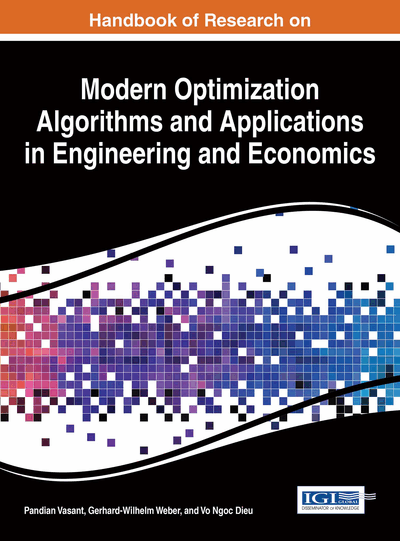# Planning of a Project with Imprecise Activity Time

Sk. Md. Abu Nayeem (Aliah University, India) and Madhumangal Pal (Vidyasagar University, India)
DOI: 10.4018/978-1-4666-9644-0.ch019

## Abstract

A project where activity times are characterized by intervals or triangular fuzzy numbers is considered in this chapter. Classical project evaluation and review technique (PERT) cannot be directly applied to such projects. Comparison of two intervals or two triangular fuzzy numbers plays an important role in this problem. Ranking methods based on some fuzzy mathematical techniques are discussed and two algorithms for finding the critical path for such a project are given. An illustrative example is also provided.
Chapter Preview
Top

## Introduction

Project evaluation and review technique (PERT) is a method to analyze different tasks required in completing a given project, especially the time needed to complete each task, and to find out the minimum time needed to complete the total project. Any individual task which utilizes resources and which has a start and an end is called an activity. The activities are generally inter-related in such a way that some activities cannot be started before the completion of some others. Thus a combination of inter-related activities in a certain order constitutes the whole project. An event represents a point in time signifying the completion of some activities and the beginning of some new ones. The inter-dependencies and the precedence relationship among the activities of a project reveal efficiently when it is represented as a network diagram. Usually, the events of a project are represented by the set of nodesof a network N= (V, E), where E is the set of edgesbetween two nodes i and j;determines the estimated time of completion of an activity within the events given by the nodes i and j.

In classical project management, the estimated completion time of each activity is taken as crisp real numbers. But, as time fluctuates with traffic conditions, payloads and so on, it is not practical to consider each activity time as a deterministic value. For example, suppose the natural completion time for the construction of a building is 17 days provided the availability of a certain number of workers and instantaneous availability of the raw materials. But, if we take into account the possibility of bad weather, the allotted time to complete the work may be say, 20 days, being there is a chance of completing it earlier. So, it will be justified to consider the time as an interval number, say, [15, 20] days or a triangular fuzzy number as (15, 17, 20) days or some other way. Different kinds of uncertainties may occur for different problems.

Management of a project where the activity times are imprecise in nature requires specialized techniques for aggregation and comparison of quantities describing the imprecision. Dubois & Prade (1980) were the first to analyze the problem for fuzzy activity time by using the extended addition/ subtraction and fuzzy maximum/ minimum for the aggregation and comparison of fuzzy quantities. The main drawback of their proposal was that the path length determined may not correspond to the original one in the network. Also the double inclusion of the uncertainties of the fuzzy durations resulted in a more fuzzy quantity during the determination of the path. This is due to the backward traversal after the extended addition has been applied in the forward calculations. Another approach to fuzzy PERT by introducing the convolution law to aggregate the fuzzy duration times was analyzed by Mares & Horak (1983) and Mares (1989). They used enumeration to examine all possible paths individually to obtain the critical path. The overall fuzzy duration times were determined in terms of maximum/ minimum elements in the supports, the modes or the median elements depending upon the decision maker’s attitude of pessimism or optimism so that the computational time can be tremendously high. Since then a lot of research in this direction have been carried out by a number of researchers by considering different kind of imprecision and different types of aggregation and comparison operations. Nayeem & Pal (2008) have studied the problem using ranking method based on ‘acceptibility index’ and deconvolution operation.

## Complete Chapter List

Search this Book:
Reset